Show TOC

###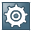Subtotal RowThis function lets you calculate and display subtotals of the preceding regular rows.

To add a subtotal row in a document, choose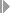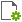(Form Settings)Table Format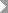and select the Visible and Active checkboxes of the option Type. The Type column appears in the relevant document. Choose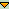and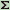.

A subtotal line summarizes all regular lines from the previous subtotal line, the first document line, or subtotals from the previous level.

• You can add a subtotal row to any line in the document.

• SAP Business One recalculates the subtotal with every change. For example, deleting a row may lead to a change in the subtotal values.

• You copy subtotal rows to target documents the same way you copy regular rows. If you have not copied the whole document; the subtotal is recalculated according to the values of the target document.

• When a subtotal row is created, the Item Description field displays Subtotal. You can modify the value if required.

• Subtotal summary amounts appear in the Total and Freight columns respectively.

• Rows of Alternative Item type are not included in the subtotal calculation.

• You can create an unlimited number of subtotal levels. However, the special design that defers one subtotal level from another is limited to the 3 levels only.

#### Example

The following table is taken from a sales quotation. The rows with no value in the Type column are regular rows that contain items.

Level

#

Type

Item No.

Quantity

Price

Total (Doc)

1

001

10

15

150

2

002

5

20

100

Level 1

3250

4

005

3

10

30

5

006

4

70

280

Level 1

6310

Level 2

7560

8

008

1

50

50

9

007

2

100

200

Level 1

10250

11

011

5

30

150

12

012

5

25

125

Level 1

13275

Level 2

14525

Level 3

151085

Rows 3 and 6 are first level subtotal rows, calculated as follows:

150 (row 1) + 100(row 2) = 250 (row 3)

30 (row 4) + 280 (row 5) = 310 (row 6)

Rows 10 and 13 are first level subtotal rows, calculated as the sum of the regular rows above them.

Row 7 is a second level subtotal row, calculated as follows:

250 (row 3) + 310 (row 6) = 560 (row 7)

Row 14 is a second level subtotal row, calculated as the sum of rows 10 and 13.

Row 15 is a third level subtotal row, calculated as follows:

560(row 7) + 525(row 14) = 1085 (row 15)